telescopeѲptics.net          ▪▪▪▪                                             CONTENTS

1.1. Point spread function (PSF)   ▐    1.3. Optical system of a telescope

# 1.2. Reflection and refraction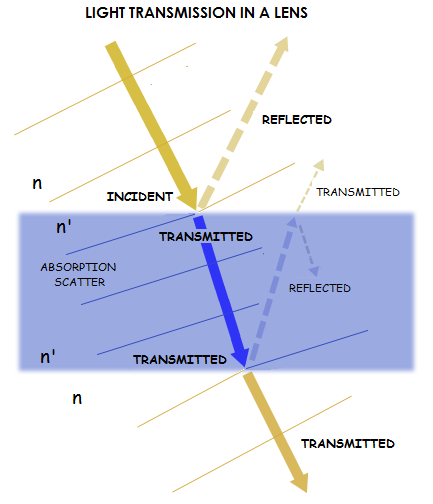In order to form images, telescopes use mirrors and lenses. Mirrors are optical surfaces that reflect light. And lenses are optical elements that transmit, i.e. refract light. Both, reflection and refraction of light result from its wave nature. In fact, mirrors and lenses both reflect and transmit light, it is only that one or the other form of response is dominant. Illustration at left shows how refracting element interacts with light; there are multiple refractions and reflections involved.

Reflection occurs when a surface exposed to light immediately emits back a portion of the energy received. As the surface atoms absorb wave energy, they become unstable, and regain stability by releasing portion of the absorbed light from their electron orbits. Due to reflection of light occurring in a precisely consistent, predictable manner, reflective surfaces can be manipulated to re-direct or re-shape optical wavefront into spherical, needed for the purpose of imaging. This can be illustrated with a linear array of three silver atoms on the mirror surface (FIG. 3 right). With the atoms being separated by ~1 Ångström (one 10-millionth of mm), the array is essentially flat. As the incident wavefront sweeps over the array, the atoms absorb wave energy and emit most of it right back. However, if the array is inclined in regard to the incident wavefront, the surface slope causes phase shift, with each successive atom's emission lagging behind by a fixed phase fraction. This in turn results in the change of orientation of the principal wavefront, so that the angle of reflection is a negative equivalent of the angle of incidence. In other words, the wavefront reflects at the angle of incidence, only at the opposite side of a normal to the surface.

Rays from the neighboring atoms are practically parallel, but they will merge and interfere in the focal zone of the mirror. As the mirror slope gradually changes along its surface, so does the slope of reflected wavefront unfolding from the mirror edge toward center. If the mirror surface has appropriate shape, the reflected wavefront will emerge spherical, with the tightest possible concentration of light energy forming around its center of curvature.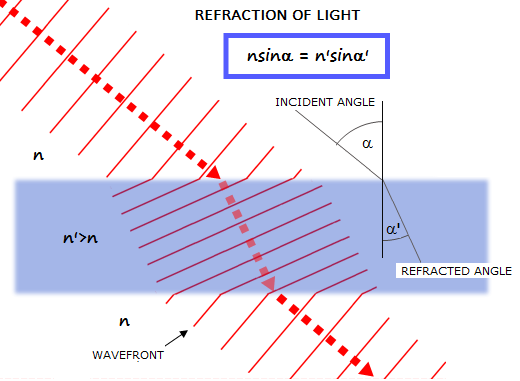Refraction of light also results from the phase shift of wavefront points, as their velocity changes within media of different optical properties (left). The ratio of velocity change is expressed by refractive index n as 1/n. Value of n spans from 1 for vacuum, to about 1.8-1.9 for the most dense commonly used optical glass types. An average refractive index of the optical crown is n~1.5, reducing the speed of light by a factor ~1/1.5 (speed of light as the speed of wave propagation, of course, doesn't change, but denser glass has more atoms in the way to excite in order to make the transmission take place, and it adds to the transmission time). Refractive index for any given media vary slightly with the wavelength of light, resulting in unequal propagation, i.e. refraction for different wavelengths - the cause of chromatic aberration.

An exaggerated section of a lens can be used to illustrate the phenomenon, mathematically described by Snell's low of refraction (FIG. 3 left). The lens surface is practically flat for a very small section, and inclined with respect to the impinging wavefront as determined by a local surface slope angle. As incident wavefront enters the glass, it slows down, while its portions still traveling through the air maintain the higher speed. This generates phase shift resulting in the change of wavefront orientation. With properly designed lens objective, these sections of refracted incident wavefront unfold into a sphere. Of course, for proper refraction, the glass has to be homogeneous, just as any other media through which light travels.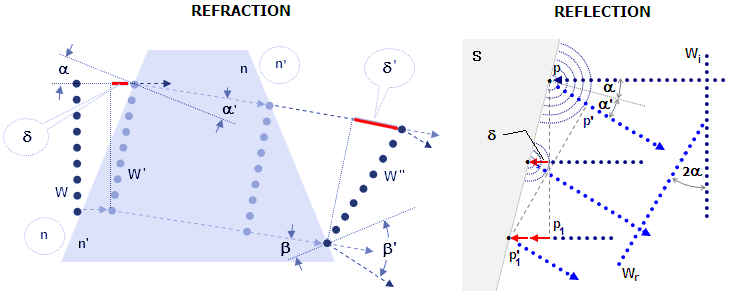FIGURE 3
: LEFT: Section of a wavefront as it travels through media of different refractive indici. The increase in phase shift δ caused by combination of surface slope and slower in-glass speed of light changes the orientation of the incoming wavefront section W into that of the refracted section W', according to the law of refraction (also: Snell's law): sines of the refracted angle α' and incident angle α  - measured from the normal to the surface - relate as the incidence index n to the index of refraction n', thus
sin
α'/sinα=n/n', or nsinα=n'sinα'.
Wavefront orientation changes again into W'' as it re-enters medium of lower refractive index. Phase shift
δ' results in the incidence angle β to change into refracted angle β'. Small angles (in radians) nearly equal their sines, which simplifies the law  to α'/α=n/n'. Setting n'=-n results in α'=-α, the law of reflection. More intuitively, the law can be grasped by looking at each dot at left as a wave in phase. As they hit glass surface, they turn it into wave emitters. Each point (atom) of glass surface hit by a wave starts re-emitting waves, mainly forward, spread in a solid angle. If we follow such wave emitted in the same direction as the incident wave, it is easy to see that these waves, coming from different points on the glass surface, have different phase in any plane perpendicular to the direction of incident light - therefore, no wavefront forms in any of those plains. In order to find the plane in which wavefront does form, we need to rotate it in in the direction of surface slope, but always less than the slope itself, since for two nearby surface emitters (i.e. surface points for which the connecting surface is practically flat) the base of triangle determining new wavefront slope (red section) relates to the base for surface slope as [(1/n)-(1/n')]/(1/n)=1-(n/n'), n and n' being the index of refraction of the medium of incidence and refraction, respectively (with 1/n and 1/n', the inverse of the refractive index, being proportional to the speed of light in two mediums). For air-to-glass surface n=1 and n' is the glass refractive index. For common crown and n'~1.5, the wavefront slope after passing from air to glass is, therefore, only about a third of the surface slope. Similar consideration applies to the refraction - i.e. wavefront reorientation - at the rear surface.
RIGHT: Reflection of light waves: flat incident wavefront section Wi, oriented vertically, strikes reflecting surface S inclined at an angle α, exciting its surface atoms. The atoms absorb and re-emit wave energy in all directions. Due to the wave emission from each next atom being delayed in time, it is also delayed by the phase fraction δ. It causes the orientation of line of points in phase change from pp1 to p'p1', as determined by the pp1p1' and pp'p1' triangle identity. As a result, reflected wavefront section Wr forms directed at an angle
α'= -α
to the surface normal, and at an angle 2α to the incident wavefront section. The minus sign indicates that this new direction forms on the opposite side of the surface normal (plane of reflection at any surface point is determined by the impinging ray and the normal). This is the law of reflection of light. As with light refraction, new wavefront cannot form in the same orientation (reversed direction) after reflection, due to the surface emitters being out of phase with respect to any plane perpendicular to the direction of incidence. It does form in a plane rotated with respect to the incident direction, and this time the slope of new wavefront is greater than that of the surface. Analogously to light refraction, the slope of the new wavefront relative to that of the surface itself is proportional to 1-(1/n'), with n'=-1 due to direction reversal in air. Thus, the slope of a small portion of wavefront for which the section of reflective surface is effectively flat, is double the slope of that section of surface itself. The form of reflected wavefront is determined by those of the incident wavefront and the surface. Proper combination of the two will produce spherical reflected wavefront. Waves emitted by atoms to directions other than that indicated by the law of reflection do not form effective wavefronts, due to the phase difference being increasing with the deviation from direction of the principal wavefront, as illustrated in Fig.1.

Local variations in glass density would cause local deformations of the wavefront, resulting in wavefront roughness.

More detailed illustration of reflection and refraction shows that the reflected wavefront, by immediately observable geometric requirement, has to have reversed symmetry with respect to the incident wavefront, due to the lineup of the points in phase after reflection being preserved only for the incident geometry rotated by 90 degrees around the axis of incidence (note that the reflected wavefront is perpendicular to the incident ray only for the 45 degree angle). In the case of refraction, the incident ray does proceed into the new medium without change in direction (dashed lines with arrows), and it's still in phase with the corresponding points of other incident rays, all lined up along the new wavefront line, but they are not orthogonal to it. Any line orthogonal to them wouldn't have them in phase anymore (phase difference between points 3 and 1' is shown with a dashed black arrow), i.e. there is no wavefront propagation in that (incident) direction. The new wavefront propagation direction is orthogonal to the orientation in which wave points line up in phase (dotted arrow).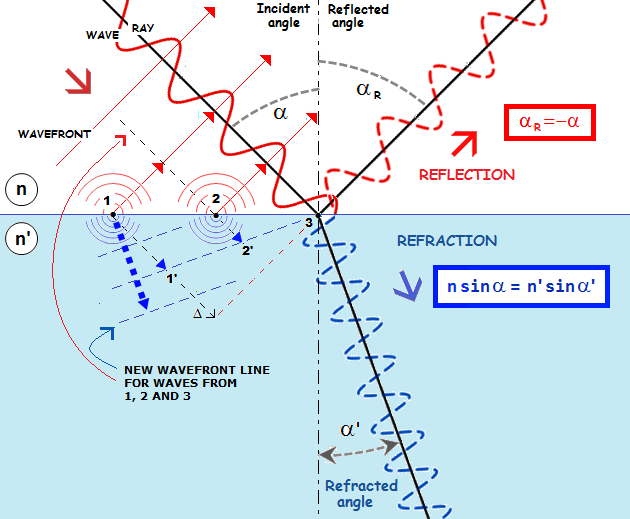It is easy to show that Snell's law applies to reflection as well. Since for reflection, index of incidence for light traveling from left to right is 1, and index of reflection is -1, substituting these values for n and n' gives law of reflection:

n'sinα' = nsinα  (law of refraction), for n=1, n'=-1 becomes law of reflection, sinα' = -sinα

Both, law of reflection and law of refraction can be derived from Fermat's principle. Some 2,000 years ago, Hero from Alexandria stated that reflected ray of light traverses the shortest possible path between two points (P and P') via reflective surface (FIG. 4A).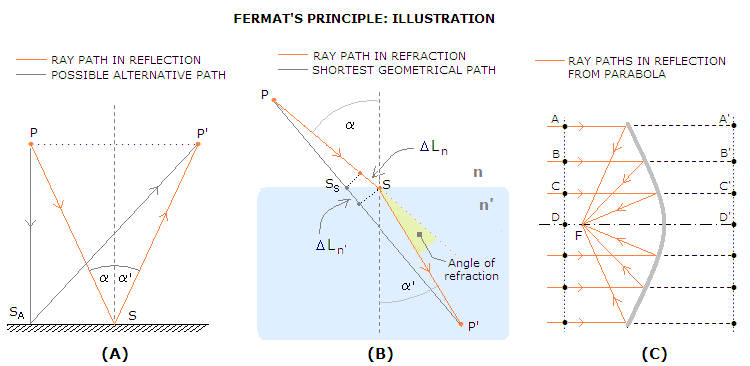FIGURE 4: A: Optical path length (OPL) for reflected ray is proportional to 2/cosα, with α=α' being the incident angle and S the point of reflection. Any alternative path is longer. For the alternative path shown, via alternative surface point SA, the OPL is, for small angles, proportional to 1+1/cos(2α). B: Optical path length for refracted ray is not the shortest possible, but the one which light transverses in the least amount of time; this, obviously, applies to reflected ray as well, thus imposes itself as the general law.

Hero's law, however, does not apply to refraction (FIG. 4B). Here, the ray path is geometrically longer than the shortest path via surface - which is a straight line connecting the two points - but light moves from point P to P' in the least amount of time via surface point S. This, of course, apply to reflection as well, and represents the basis of the general principle defined by Fermat, which states that

light ray traversing two given points follows the path that requires the least amount of time,

i.e. one of the shortest optical path length (shown is ray refracted at air/glass surface, with the refractive index n'~1.5; path differential in air ΔLn is geometrically somewhat larger than that in glass, ΔLn', but less than 50%, which is how much more time light needs in the glass vs. air to span any given distance).

Depending on optical surface, there can be more than a single path satisfying Fermat's principle. A ray traversing the two foci via reflective ellipsoidal surface will have an indefinite number of alternative paths requiring the same minimum amount of time - we could say that these rays have no other choice. When the second focus is at infinity, optical path length from any point on the incident wavefront (A, B, C, D...) to the focus point F is constant at the minimum stationary value. For such surface, there is a straight line whose point (A', B', C', D'...) to surface separation equals the corresponding separation from surface to a fixed point (F) - the definition of a parabola (FIG. 4C).